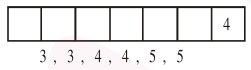# A seven digit number is`
Question:

A seven digit number is formed using digits 3 , $3,4,4,4,5,5$. The probability, that number so formed is divisible by 2 , is :

1. $\frac{6}{7}$

2. $\frac{1}{7}$

3. $\frac{3}{7}$

4. $\frac{4}{7}$

Correct Option: , 3

Solution:

Digits $=3,3,4,4,4,5,5$

Total 7 digit numbers $=\frac{7 !}{2 ! 2 ! 3 !}$

Number of 7 digit number divisible by 2 $\Rightarrow$ last digit $=4$Now 7 digit numbers which are divisible by 2

$=\frac{6 !}{2 ! 2 ! 2 !}$

Required probability $=\frac{\frac{6 !}{2 ! 2 ! 2 !}}{\frac{7 !}{3 ! 2 ! 2 !}}=\frac{3}{7}$